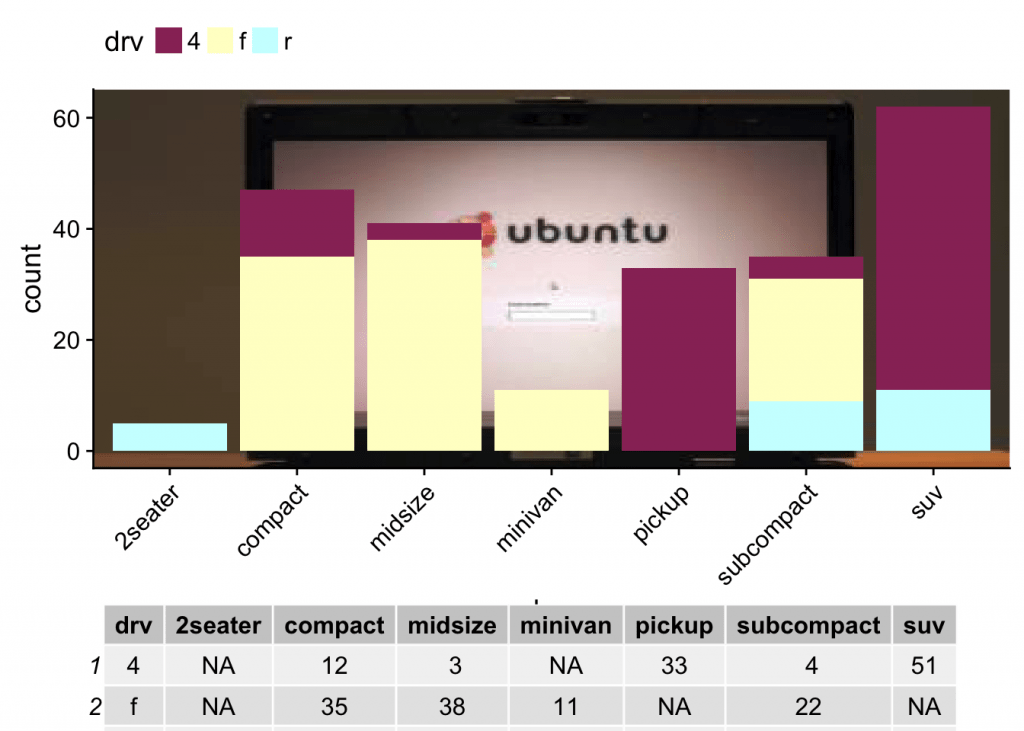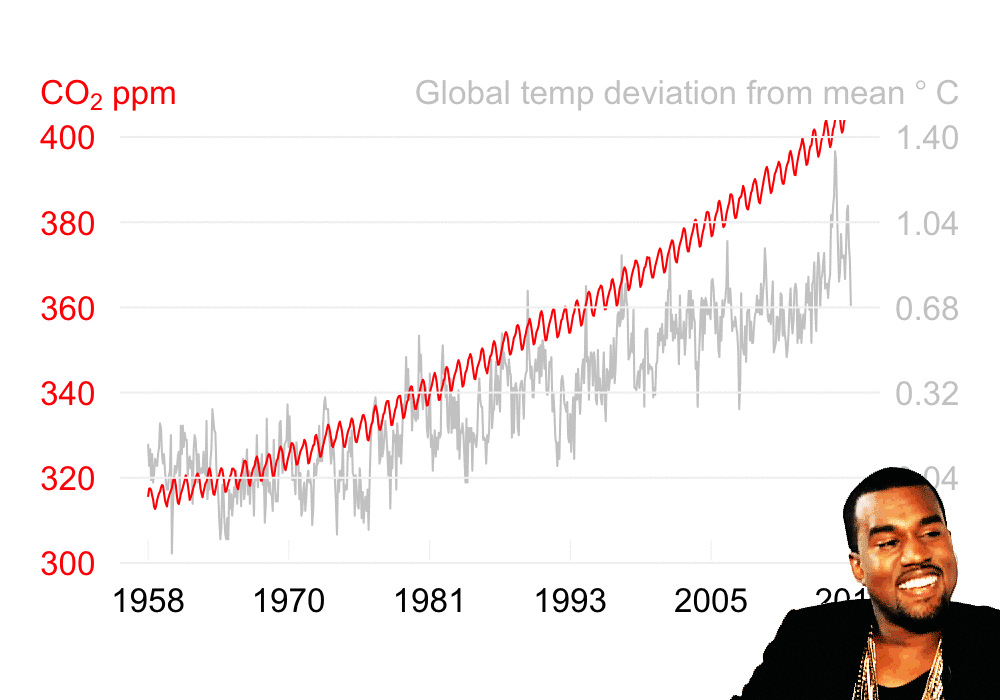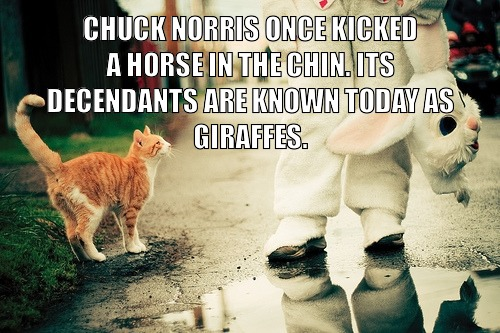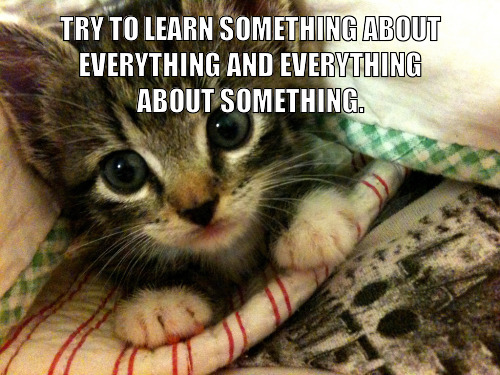Want to share your content on R-bloggers? click here if you have a blog, or here if you don't.

Yes, I know. I’m a hypocrite to be creating ‘interesting’ stuff using `R`. If you’ll excuse me just this one time, you’ll see some silly fun using `R`. It is an experiment to see how much we can extend `R` as well as learning new packages.

# Add a background image to a plot

I don’t know when you would need to use this, but if you ever find a need to add a background image in a `ggplot`, you can use this following function called `sighr`.

```require(ggplot2)
require(jpeg)
require(grid)
require(gridExtra)
require(dplyr)
require(tidyr)
require(cowplot)

#ggthemes Excel theme colors
sigh_colors <- c("#993366", "#FFFFCC", "#CCFFFF", "#660066", "#FF8080", "#0066CC", "#CCCCFF")

sighr <- function(df = mpg, xvar = 'class', fillvar = 'drv', sighmore = FALSE) {
img_url <- 'http://lorempixel.com/400/200'
tmp_file <- tempfile()
file.remove(tmp_file)

rstr <- rasterGrob(img, width = unit(1,"npc"), height = unit(1,"npc"), interpolate = FALSE)

g <- ggplot(data = df)  + annotation_custom(rstr, -Inf, Inf, -Inf, Inf)
g <- g + geom_bar(aes_string(x = xvar, fill = fillvar))
g <- g + theme(legend.position = "top", legend.background = element_rect(color = "blue"),
panel.grid = element_line(size = rel(4), color = "purple"),
axis.text.x = element_text(angle = 45, hjust = 1))
g <- g + scale_fill_manual(values = sigh_colors)

if (sighmore){
totals <- group_by_(df, xvar, fillvar) %>% count() %>% spread_(xvar, 'n')
total_grob <- tableGrob(totals)
g <- plot_grid(g, total_grob, nrow = 2, rel_heights = c(7/8, 1/8))
}
return (g)
}```

Executing this function we get a random background image and bonus: Excel 95 color scheme and turned axis labels.

`sighr()`If you pass the additional argument `sighmore = TRUE`, you get a table attached with the plot.

`sighr(sighmore = TRUE)`# Create a gif

GIFs actually could be useful depending on your use. Here, I wanted to combine a chart with carbon emissions data and global temperature change with a “I-don’t-believe-it” gif.

Here we go.

## First, let’s load all the good libraries.

```library(readr)
library(lubridate)
library(dplyr)
library(tidyr)
library(magick)```

```##download CO2 emissions data
mo2Num <- function(x) match(tolower(x), tolower(month.abb))
comment = "#",
col_names = c('year', 'month', 'dec_date', 'avg', 'interpolated', 'trend', 'days'))
co2ppm <- mutate(co2ppm, date = as.Date(date_decimal(decimal = dec_date)))

skip = 1, na = '*****',
col_types = cols(.default = 'd', Year = "i"))
global_temp_l <- select(global_temp, 1:13) %>% gather(key = month, value = temp, -Year)
global_temp_l <- mutate(global_temp_l, date = ymd(paste(Year, month, 1, sep = "-")),
month_number = mo2Num(month))

temp_co2ppm <- inner_join(select(co2ppm, year, month, interpolated),
select(global_temp_l, Year, month_number, temp),
by = c("year" = "Year", "month" = "month_number")) %>% mutate(date = ymd(paste(year, month, 1, sep = "-")))

temp_co2ppm_l <- gather(temp_co2ppm, key = what, value = val, -year, -month, -date) %>%
mutate(ifelse(what == 'interpolated', 'CO2ppm', ifelse(what == 'temp', 'deviation temp', NA)))```

## Plot the data

You will see I’m not using `ggplot` here. I had a difficult time getting two y-axis going. `base-R` graphics to rescue.

```png(filename = "co2_temp_plot.png", width = 5, height = 3.5, units = "in", res = 200)
par(mar = rep(3, 4), las = 1)
with(temp_co2ppm, plot(x = date, y = temp, type = 'l', col = "grey80", axes = FALSE, xlab = NA, ylab = NA, ylim = c(-.4, 1.4)))
axis(side = 4, at = seq(from = -.40, to = 1.40, length.out = 6), tick = FALSE, hadj = 1, line = 1, col.axis = "grey80")
par(new = TRUE)
with(temp_co2ppm, plot(x = date, y = interpolated, type = 'l', col = "red", axes = FALSE, ylim = c(300, 400)))
abline(h = seq(from = 300, to = 400, length.out = 6), col = "grey95")
axis(side = 2, at = seq(from = 300, to = 400, length.out = 6), tick = FALSE, line = 1, hadj = 0, col.axis = "red")
axis.Date(side = 1, at = seq.Date(from = min(temp_co2ppm\$date), to = max(temp_co2ppm\$date), length.out = 6),
lwd = 0, line = -1, format = "%Y", lwd.ticks = 0.5, col.ticks = "grey95")
mtext(expression(""*CO*" ppm"), side = 2, col = "red", at = 410, adj = 0, line = 2)
mtext(expression("Global temp deviation from mean"~degree~C), side = 4, col = "grey80", at = 410, adj = 1, line = 2)
dev.off()```

## Read the plot, gif, and combine

Using the `magick` library, we can read the saved plot as well as the gif and write a new combined gif.

```bkgrnd_plot <- image_read("co2_temp_plot.png")

frames <- lapply(gif_raw, function(frame) {
image_composite(bkgrnd_plot, frame, offset = "+650+350")
})

plot_gif <- image_animate(image_join(frames), fps = 20)
image_write(plot_gif, "co2plot_kanye.gif")```# Generate cat memes

This was truly fun and I was surprised by the results. Some were really on point. To make this code work, you will need to get an API key from [. This function downloads a random quote based on your select: Chuck Norris, Ron Swanson, or a motivational quote.

```library(magick)
library(dplyr)
library(magrittr)
library(httr)
library(jsonlite)
library(stringr)```

`hd_key <- c('X-Mashape-Key' = 'get-your-own-key') `

## Create a function to wrap the API call

```api_text <- function(url, extra = ""){
return (fromJSON(rawToChar(cntt\$content)))
}```

## Create the meme

This function will change the font type to Impact and adds the quote to a random cat photo from the site thecatapi.com. By default, the function will use Ron Swanson quote.

```cat_meme <- function(which_quote = 5){
if (which_quote == 2 ){
cat_text <- api_text('https://andruxnet-random-famous-quotes.p.mashape.com/?cat=famous&count=1', extra = hd_key)\$quote
}else if (which_quote == 3 ){
cat_text <- api_text('https://matchilling-chuck-norris-jokes-v1.p.mashape.com/jokes/random?category=animal', extra = hd_key)\$value
}else {
cat_text <- api_text('http://ron-swanson-quotes.herokuapp.com/v2/quotes')
}
cat_text <- str_to_upper(str_wrap(cat_text, width = 30))
image_annotate(cat_img, cat_text, gravity = "north", size = 30, location = "+0+10", color = "white", font = 'impact', strokecolor = "black")
}```

Let’s try it:

`cat_meme()`With Chuck Norris this time:

`cat_meme(which_quote = 3)``cat_meme(which_quote = 2)`# Create a motivational quote Twitter bot

The cat meme function gave me the idea to create a Twitter bot that:

• pulls a motivational quote from www.mashape.com
• gets a random image from unsplash.com
• blurs the image
• combines the image and the quote
• posts the combined image to Twitter

Of course, for this to succeed, you need a Twitter account and a Twitter API key and token.

```library(twitteR)
library(stringr)```

```setup_twitter_oauth(consumer_key = "your-consumer_key",
consumer_secret = "your-consumer_secret",
access_token = "your-access_token",
access_secret = "your-access_secret")```

`nand_logo <- image_read('yourlogo.png')`

## Create the quote function

```motivational_qt <- function(){
bkgrnd_img <-  image_read('https://unsplash.it/800/500/?random') #get a random image from unsplash
bkgrnd_img <- image_blur(bkgrnd_img, radius = 6, 5) #blur the image
bkgrnd_img <- image_colorize(image = bkgrnd_img, color = "grey90", opacity = 40) #add a grey layer for better reading of the text
quote <- api_text('https://andruxnet-random-famous-quotes.p.mashape.com/?cat=famous&count=1', extra = hd_key) # get a quote
quote_a <- paste0(str_wrap(quote\$quote, width = 40), "\n - ", quote\$author) #wrap the text to make it fit on the image
qt_img <- image_annotate(bkgrnd_img, quote_a, gravity = "center", size = 30, location = "+0+0", color = "#A13700", font = '/System/Library/Fonts/Noteworthy.ttc', strokecolor = "#A13700") #add the text to the image
qt_logo <- image_composite(qt_img, image_scale(nand_logo, "30%"), operator = "atop", offset = "+730+450") #add the logo
qt_logo_site <- image_annotate(qt_logo, "www.nandeshwar.info", gravity = 'southwest', location = "+10+10", color = "white", size = 15, font = 'arial') #add the url
t <- image_write(image = qt_logo_site, path = "quote_text.png") #save the image
return(quote_a) #return text
}```

Let’s try the function

```motivational_qt()
##  ""The artist is nothing without the gift,\nbut the gift is nothing without work.\n - Emile Zola""```

If everything worked, this function should return a quote and the image should be saved locally.This is the easiest part. Just get the text from the `motivational_qt()` function and the image and send it to Twitter. Please use caution and don’t spam.

```updateStatus(text = str_sub(paste0("QuoteBot courtesy @n_ashutosh @opencpu @unsplash @geoffjentry ",
str_replace(motivational_qt(), pattern = "\n", replacement = " ")),
end = 140),
mediaPath = "quote_text.png")```
That’s it. I hope you enjoy having some fun with `R` – but please, please use all of this very responsibly.

The post Little silly fun with R appeared first on nandeshwar.info.